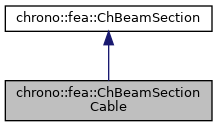chrono::fea::ChBeamSectionCable Class Reference

## Description

Simplified geometry for a 'cable' beam section in 3D, that is a beam without torsional stiffness and with circular section (i.e.same Ixx and Iyy properties).

This material can be shared between multiple beams.

#include <ChBeamSection.h>

Inheritance diagram for chrono::fea::ChBeamSectionCable:[legend]
Collaboration diagram for chrono::fea::ChBeamSectionCable:[legend]

## Public Member Functions

void SetArea (const double ma)
Set the cross sectional area A of the beam (m^2)

double GetArea () const

void SetI (double ma)
Set the I moment of inertia of the beam (for flexion about y axis or z axis) Note: since this simple section assumes circular section, Iyy=Izz=I.

double GetI () const

void SetDiameter (double diameter)
Shortcut: set Area and I inertia at once, given the diameter of the beam assumed with circular shape. More...

void SetDensity (double md)
Set the density of the beam (kg/m^3)

double GetDensity () const

void SetYoungModulus (double mE)
Set E, the Young elastic modulus (N/m^2)

double GetYoungModulus () const

void SetBeamRaleyghDamping (double mr)
Set the Rayleigh damping ratio r (as in: R = r * K ), to do: also mass-proportional term.

double GetBeamRaleyghDamping ()Public Member Functions inherited from chrono::fea::ChBeamSection
void SetDrawThickness (double thickness_y, double thickness_z)
Sets the rectangular thickness of the beam on y and z directions, only for drawing/rendering purposes (these thickness values do NOT have any meaning at a physical level, use ChBeamSectionBasic::SetAsRectangularSection()

double GetDrawThicknessY ()

double GetDrawThicknessZ ()

bool IsCircular ()
Tells if the section must be drawn as a circular section instead than default rectangular.

void SetCircular (bool ic)
Set if the section must be drawn as a circular section instead than default rectangular.

void SetDrawCircularRadius (double draw_rad)
Sets the radius of the beam if in 'circular section' draw mode, only for drawing/rendering purposes (this radius value do NOT have any meaning at a physical level, use ChBeamSectionBasic::SetAsCircularSection()

double GetDrawCircularRadius ()

## Public Attributes

double Area

double I

double E

double density

double rdampingPublic Attributes inherited from chrono::fea::ChBeamSection
double y_drawsize

double z_drawsize

bool is_circular

## Member Function Documentation

 void chrono::fea::ChBeamSectionCable::SetDiameter ( double diameter )

Shortcut: set Area and I inertia at once, given the diameter of the beam assumed with circular shape.